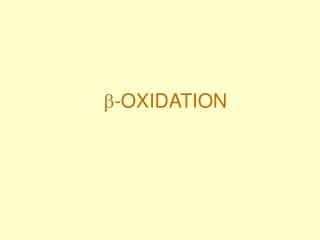DownloadDownload Presentation-OXIDATION

# -OXIDATION

Download Presentation## -OXIDATION

- - - - - - - - - - - - - - - - - - - - - - - - - - - E N D - - - - - - - - - - - - - - - - - - - - - - - - - - -
##### Presentation Transcript

1. -OXIDATION

2. courses.cm.utexas.edu/.../FinalReview.html

3. Long chain acyl-CoA dehydrogenase: removes two hydrogens by the reduction of FAD to FADH2 converting the single C  C bond to a double C    C  bond. The FADH2 reacts with the Respiratory Chain allowing oxidative phosphorylation to generate ATP. courses.cm.utexas.edu/.../FinalReview.html

4. Hydration: H and OH of water is added across the double bond formed by the removal (above) of two hydrogens to FADH2. courses.cm.utexas.edu/.../FinalReview.html

5. Dehydrogenation #2 : here again two hydrogens are removed and added to NAD+ to form H+ and NADH which reacts with the Respiratory Chain allowing oxidative phosphorylation to generate ATP. courses.cm.utexas.edu/.../FinalReview.html

6. Thiolysis: Coenzyme A (SCoA) cleaves the fatty acyl-CoA between the beta and alpha carbons, splitting the two-carbon acetyl-CoA and the fatty acyl-CoA shorted by two carbons. courses.cm.utexas.edu/.../FinalReview.html

7. www.steve.gb.com/science/molecules.html

8. www-medlib.med.utah.edu/NetBiochem/tabletit.htm

9. courses.cm.utexas.edu/.../FinalReview.html

10. Example: oxidation of 16 C fatty acid palmitoyl-CoA Palmitoyl-CoA + 7 CoASH + 7 FAD + 7 NAD+ + 7 H2O —> 8 AcetylCoA + 7 FADH2 + 7 NADH + 7 H+ Overall reaction, along with downstream oxidation of acetyl-CoA in TCA cycle: Acetyl-CoA + 2 O2 + 10 Pi + 10 ADP —> CoA + 10 ATP + 2 H2O + 2 CO2 16 C palmitate fatty acyl-CoA requires 7 turns of the fatty acid spiralto produce: 8 Acetyl-CoA x 12        =    96 ATP (3NADH + 1FADH2 + 1 GTP = 9 + 2 =12) 7 NADH x 3               = 21 ATP 7 FADH2 x 2              = 14 ATP 131 =  131 ATP - 2 (activation) = Net 129 ATP Standard free energy change for oxidation of palmitate to CO2 + H2O = 9800 kJ/mol ATP x 30.5 kJ/mol

11. Figure this out…….. • The net result of the oxidation of one mole of oleic acid (an 18-carbon fatty acid) will be 146 moles of ATP (2 mole equivalents are used during the activation of the fatty acid), as compared with 114 moles from an equivalent number of glucose carbon atoms.

12. Calculate the total ATP produced from the complete metabolism of stearic acid, CH3(CH2)16COOH   Follow steps below. a. Calculate number of acetyl CoA.   b. Calculate number of turns for the fatty acid spiral and citric acid cycle. c. Calculate ATP per turn of the fatty acid spiral if FAD and NAD+ enter the ETC   d. Calculate the total ATP for the fatty acid spiral.   e. Calculate the total ATP per turn of the citric acid cycle using a diagram of the cycle.   f. Calculate the total ATP from the citric acid cycle g. Calculate the grand total of ATP